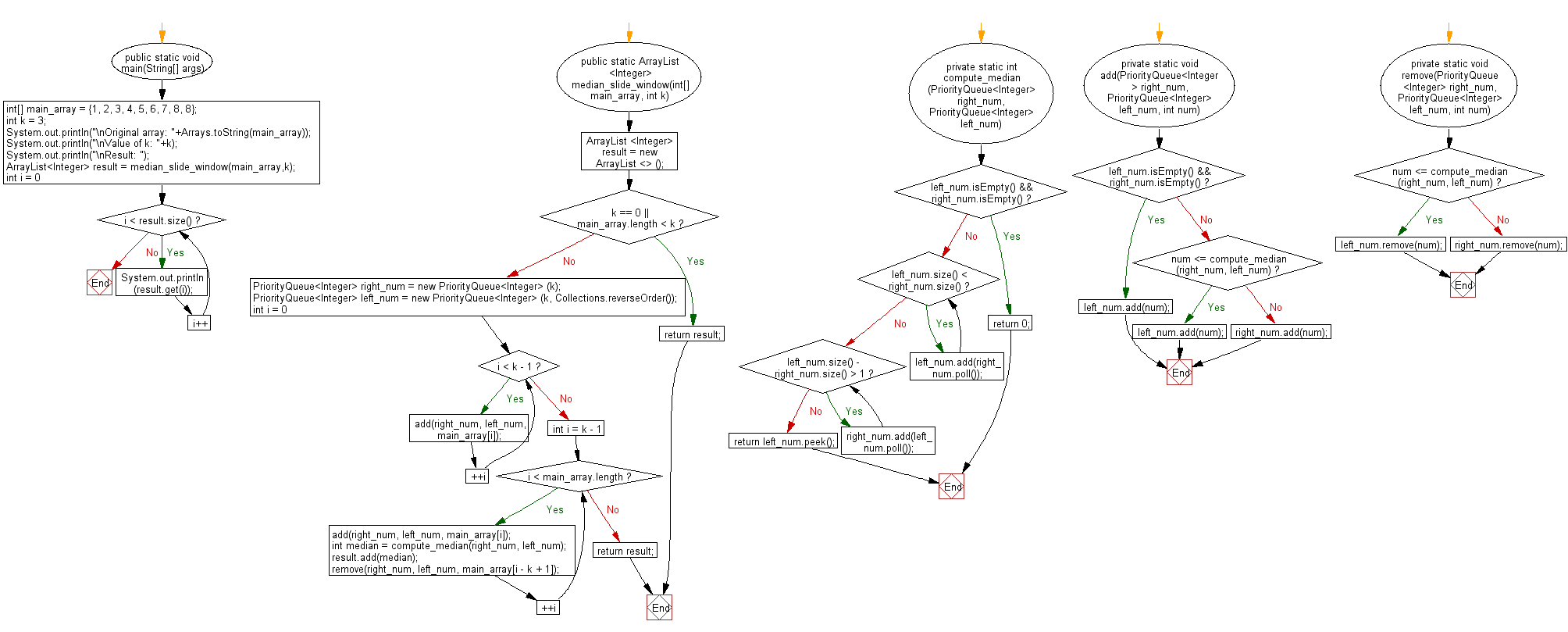﻿ Java programming exercises: Find the median of the number inside the window - w3resource# Java Exercises: Find the median of the number inside the window

## Java Basic: Exercise-173 with Solution

Write a Java program to find the median of the number inside the window (size k) at each moving in a given array of integers with duplicate numbers. Move the window from the start of the array.

{|1, 2, 3|, 4, 5, 6, 7, 8, 8} -> Return median 2
{1, |2, 3, 4|, 5, 6, 7, 8, 8} -> Return median 3
{1, 2, |3, 4, 5|, 6, 7, 8, 8} -> Return median 4
{1, 2, 3, |4, 5, 6|, 7, 8, 8} -> Return median 5
{1, 2, 3, 4, |5, 6, 7|, 8, 8} -> Return median 6
{1, 2, 3, 4, 5, |6, 7, 8|, 8} -> Return median 7
{1, 2, 3, 4, 5, 6, |7, 8, 8|} -> Return median 8
Result array {2, 3, 4, 5, 6, 7, 8}

Sample Solution:

Java Code:

``````import java.util.*;
import java.util.Arrays;

public class Solution {
public static void main(String[] args) {
int[] main_array = {1, 2, 3, 4, 5, 6, 7, 8, 8};
int k = 3;
System.out.println("\nOriginal array: " + Arrays.toString(main_array));
System.out.println("\nValue of k: " + k);
System.out.println("\nResult: ");
ArrayList < Integer > result = median_slide_window(main_array, k);
for (int i = 0; i < result.size(); i++) {
System.out.println(result.get(i));
}
}
public static ArrayList < Integer > median_slide_window(int[] main_array, int k) {
ArrayList < Integer > result = new ArrayList < > ();
if (k == 0 || main_array.length < k) {
return result;
}
PriorityQueue < Integer > right_num = new PriorityQueue < Integer > (k);
PriorityQueue < Integer > left_num = new PriorityQueue < Integer > (k, Collections.reverseOrder());

for (int i = 0; i < k - 1; ++i) {
}

for (int i = k - 1; i < main_array.length; ++i) {
int median = compute_median(right_num, left_num);
remove(right_num, left_num, main_array[i - k + 1]);
}
return result;
}

private static int compute_median(PriorityQueue < Integer > right_num, PriorityQueue < Integer > left_num) {
if (left_num.isEmpty() && right_num.isEmpty()) {
return 0;
}
while (left_num.size() < right_num.size()) {
}

while (left_num.size() - right_num.size() > 1) {
}
return left_num.peek();
}

private static void add(PriorityQueue < Integer > right_num, PriorityQueue < Integer > left_num, int num) {
if (left_num.isEmpty() && right_num.isEmpty()) {
return;
} else {
if (num <= compute_median(right_num, left_num)) {
} else {
}
}
}

private static void remove(PriorityQueue < Integer > right_num, PriorityQueue < Integer > left_num, int num) {
if (num <= compute_median(right_num, left_num)) {
left_num.remove(num);
} else {
right_num.remove(num);
}
}
}
```
```

Sample Output:

```Original array: [1, 2, 3, 4, 5, 6, 7, 8, 8]

Value of k: 3

Result:
2
3
4
5
6
7
8
```

Pictorial Presentation:Flowchart:Java Code Editor:

Company:

What is the difficulty level of this exercise?

﻿

## Java: Tips of the Day

Count the number of occurrences of a char in a String:

```int count = StringUtils.countMatches("a.b.c.d", ".");
```

Ref: https://bit.ly/2OTy6sG